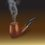# Question on decimal number system

Let me check how many of our mathematics enthusiasts here have given a thought to this question. We know that our decimal number system consists of the ten digits, viz., 0, 1, 2, 3, 4, 5, 6, 7, 8, 9. We start our counting with ZERO(0), then we count as following:

ONE, TWO, THREE, FOUR, FIVE, SIX, SEVEN, EIGHT, NINE..

Now the next number is TEN(10). The very peculiar thing about this number is that in our decimal system (i.e., common man's number system) we write this number as a 1 followed by a 0. Then comes ELEVEN(11), written as a 1 followed by a 1, then TWELVE(12) written as a 1 followed by a 2, and so on. Now my question is,why is this so? Instead of writing TEN as 10, we could have written it as 1 followed by two 0's, i.e., as '100', or 0 followed by 1, i.e., as '01' or in any other way. Same argument applies in the case of ELEVEN, TWELVE, and so on. My question may seem to be an idiotic one or a meaningless one, but if you have a mathematical insight, then you will realize that it is indeed a very intriguing one. Try to account for this peculiar way in which our ancestors had designed a number system which has framed the structure of the whole of modern science and technology.Note by Kuldeep Guha Mazumder
6 years ago

This discussion board is a place to discuss our Daily Challenges and the math and science related to those challenges. Explanations are more than just a solution — they should explain the steps and thinking strategies that you used to obtain the solution. Comments should further the discussion of math and science.

When posting on Brilliant:

• Use the emojis to react to an explanation, whether you're congratulating a job well done , or just really confused .
• Ask specific questions about the challenge or the steps in somebody's explanation. Well-posed questions can add a lot to the discussion, but posting "I don't understand!" doesn't help anyone.
• Try to contribute something new to the discussion, whether it is an extension, generalization or other idea related to the challenge.

MarkdownAppears as
*italics* or _italics_ italics
**bold** or __bold__ bold
- bulleted- list
• bulleted
• list
1. numbered2. list
1. numbered
2. list
Note: you must add a full line of space before and after lists for them to show up correctly
paragraph 1paragraph 2

paragraph 1

paragraph 2

[example link](https://brilliant.org)example link
> This is a quote
This is a quote
    # I indented these lines
# 4 spaces, and now they show
# up as a code block.

print "hello world"
# I indented these lines
# 4 spaces, and now they show
# up as a code block.

print "hello world"
MathAppears as
Remember to wrap math in $$ ... $$ or $ ... $ to ensure proper formatting.
2 \times 3 $2 \times 3$
2^{34} $2^{34}$
a_{i-1} $a_{i-1}$
\frac{2}{3} $\frac{2}{3}$
\sqrt{2} $\sqrt{2}$
\sum_{i=1}^3 $\sum_{i=1}^3$
\sin \theta $\sin \theta$
\boxed{123} $\boxed{123}$

Sort by:

There are quite a few different number systems, but the most common ones used in mathematics are integer base positional number systems. This is jargon for saying that all numbers are expressed as follows, given integer base $n$ and digits ${ a }_{ i }$

$\displaystyle \sum _{ i=1 }^{ \infty }{ { a }_{ i }{ n }^{ i-1 } }$

One useful property this has, for integer bases, is that for each number, there is an unique representation written in this form. This isn't necessarily the case for non-integer bases or other positional numbering systems.

Having said that, our decimal number system is base $10$, and uses digits $0,1,2,3,4,5,6,7,8,9$. Note that for any integer base, the number of the digits is equal to the integer base, and thus the base number itself cannot be represented by any of them. If $""$ is a digit itself, then we are talking about base $11$, and we end up with the sequence

$0,1,2,3,4,5,6,7,8,9,,10, 11, 12, 13, 14, 15, 16, 17, 18, 19, 1, 20, 21,...$

as awkward as that is, which is why nobody uses that.

- 6 years ago

Yes yes..well, that is why the decimal number system find its place above the non-positional number systems like the Roman one, in the list of convenience of use. But I have a very nice way of explaining the concept to young minds..